## SQL Server 2000

#### Course at a glance

Included in these subscriptions:

• Dev & IT Pro Video
• Dev & IT Pro Power Pack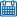Release date 2/3/2004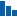Level Intermediate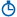Runtime 22h 24m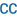Closed captioning N/A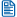Transcript N/A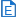eBooks / courseware Included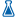Hands-on labs Included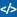Sample code Included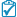Exams Included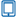Platform Internet Explorer Only#### Course description

This course is for developers coming from Microsoft Access, Visual Basic, Active Server Pages or other development backgrounds who want to learn to build successful databases solutions with SQL Server. Even developers who have already used Oracle, Sybase, DB2 or other relational database management systems will find this course useful for getting up to speed on SQL Server 2000. Learn about the features that are available in SQL Server; how to design and create a database; and how to build basic queries using Transact-SQL, the language of SQL Server. Then, you will learn how to build effective views, stored procedures, triggers, and user-defined functions, using Transact-SQL. You will also learn about the advanced features available to you for maintaining, analyzing, searching and distributing your data. Integrate your data safely with Internet and cross-platform applications.

#### Meet the expertsAndy Baron is a nationally recognized industry expert specializing in Visual Basic, Visual C#, ASP.NET, ADO.NET, SQL Server, and SQL Server Business Intelligence. He is an experienced consultant, author, and editor for several industry publications and speaker at industry conferences. Andy has also been repeatedly selected as a Microsoft MVP for his contributions to Microsoft's online support.Mary Chipman is a programmer-writer for the Data Access Technologies team at Microsoft. Previously, she was a senior consultant and was repeatedly recognized as a Microsoft MVP. Mary is the co-author of the "Microsoft Access Developer's Guide to SQL Server" (Sams) and has written additional books and articles for technical journals. She has been a frequent speaker at industry conferences and is an experienced trainer and courseware author.

#### Module 1

##### Editions and Graphical Tools (12:42)
• Introduction (00:44)
• SQL Server Editions (02:21)
• Pricing and Licensing (00:47)
• SQL Server Tools (04:54)
• Enterprise Manager (01:10)
• Enterprise Wizards (00:58)
• Summary (00:22)
##### Enterprise Manager (17:21)
• Databases (00:31)
• Server Node (02:50)
• Transformation Services (00:39)
• Management (02:58)
• Replication (00:32)
• Security (01:48)
• Support Services (00:55)
• Meta Data Services (00:33)
• Books Online (05:55)
• Summary (00:39)
##### Installing SQL Server (17:36)
• Instances (00:30)
• Multiple Instance (01:54)
• Requirements (02:35)
• Backward Compatibility (02:07)
• Begin Installation (03:34)
• Select Components (02:35)
• Finish Installation (01:55)
• Summary (00:22)
##### Configuring SQL Server (09:41)
• Introduction (00:32)
• EnterpriseManager (00:41)
• Registration Properties (01:19)
• Properties (02:32)
• Connections (01:20)
• Server Settings (01:27)
• Database Settings (01:26)
• Summary (00:22)
##### Relational Database Design (22:41)
• Overview (00:51)
• Organizing Facts (01:21)
• Key Types (03:19)
• Data Normalization (01:01)
• Query Analyzer (03:13)
• First Normal Form (03:21)
• Second Normal Form (02:06)
• Third Normal Form (02:57)
• Summary (00:55)
##### Defining Relationships (12:49)
• Introduction (01:26)
• Diagrams (01:21)
• One-to-Many (01:13)
• Many-to-Many (01:51)
• One-to-One (03:56)
• Limiting Values (01:52)
• Summary (01:09)

#### Module 2

##### Creating Databases (23:55)
• Introduction (00:26)
• Physical Storage (02:21)
• Files/Filegroups (03:26)
• SQL Profiler (02:44)
• Create a Database (05:24)
• Create with Analyzer (03:10)
• Set Properties (00:56)
• Recovery Models (05:01)
• Summary (00:27)
##### Tables and Data Types (20:20)
• Introduction (00:22)
• Begin Table Creation (01:25)
• Character (02:34)
• Numeric (02:08)
• Fractional Numbers (03:11)
• Scaled Integers (03:34)
• Binary (01:02)
• Identifier (02:23)
• Timestamp (01:28)
• Sql_variant (01:48)
• Summary (00:24)
##### Refining Table Definitions (24:22)
• Introduction (00:36)
• Entered Values (03:44)
• Computed Values (03:49)
• Constraints (01:50)
• Foreign Key Constraint (01:59)
• Create a Relationship (03:49)
• Diagram Views (04:02)
• Table Views (00:57)
• Summary (00:58)
##### Retrieving Data (25:03)
• Introduction (01:08)
• Attach Database (01:19)
• Open Script File (01:47)
• Execute Select Queries (02:12)
• Concatenating (01:47)
• Aliasing (02:47)
• Select/Select Distinct (01:01)
• Where Clause (00:48)
• Like Operator (03:45)
• Between Operator (00:41)
• Null Values (01:23)
• And/Or/Not (03:09)
• In Operator (01:14)
• Summary (00:42)

#### Module 3

##### Sorting and Grouping (13:13)
• Introduction (01:01)
• Order By (02:03)
• Counting (02:00)
• Group By (02:12)
• Having (02:56)
• Top (02:01)
• Summary (00:59)
##### Joining Tables (26:28)
• Introduction (00:34)
• View Diagram (02:31)
• Cross Join (01:38)
• Joining with Where (00:58)
• Inner Join (02:48)
• Multiple Table Join (01:22)
• Create a View (04:08)
• Left Outer Join (03:52)
• Right Outer Join (01:19)
• Full Join (00:32)
• Combining Joins (02:23)
• Change Join Type (01:18)
• Self Join (02:24)
• Summary (00:40)
##### Inserting Data (18:19)
• Introduction (00:51)
• Analyzer Setup (00:47)
• Insert a row (01:36)
• Identity Values (02:49)
• Delimiters (01:55)
• Insert Multiple Rows (01:15)
• Select Into (01:55)
• Create Temp Table (04:40)
• Bulk Insert (01:51)
• Summary (00:39)
##### Updating and Deleting (06:06)
• Update a Row (00:28)
• Update Multiple Rows (02:03)
• Update Another Table (01:34)
• Delete Rows (00:35)
• Truncate Command (01:08)
• Summary (00:18)

#### Module 4

##### Using the Query Analyzer (14:10)
• Introduction (00:36)
• Customize Toolbar (04:08)
• Go To Line, Bookmarks (00:55)
• Templates (03:44)
• Summary (00:05)
##### Sets and Procedures (14:09)
• Introduction (00:23)
• Execution and Result Sets (04:27)
• Object Browser (03:41)
• Stored Procedures (05:32)
• Summary (00:05)
##### Files Tools and Options (17:01)
• Introduction (00:29)
• Object Search (03:15)
• Manage Indexes (02:11)
• Manage Statistics (01:01)
• Options (03:18)
• Connection Options (02:29)
• Window & Help Menus (03:40)
• Summary (00:37)
##### Programming with T-SQL (19:36)
• Introduction (01:11)
• Batches (03:52)
• DECLARE Variables (04:54)
• Using CAST and CONVERT (08:07)
• Using STR (01:26)
• Summary (00:05)
##### Functions and Commands (14:54)
• Introduction (00:50)
• Built-in Functions (00:45)
• Working with NULLs (03:47)
• COALESCE (02:52)
• ISNUMERIC (01:32)
• RAND (02:10)
• ROUND (02:29)
• Summary (00:28)
##### Built-in T-SQL Functions (13:44)
• Introduction (00:37)
• REPLACE & STUFF (03:53)
• LEN, LEFT & RIGHT (03:22)
• CHARINDEX (01:33)
• SPACE (01:40)
• CHAR & ASCII (00:18)
• LOWER & UPPER (01:17)
• LTRIM & RTRIM (00:59)
• Summary (00:05)
##### More T-SQL Functions (17:20)
• Date & Time Functions (00:38)
• DATEPART (02:48)
• DATEFIRST (01:55)
• @@ROWCOUNT (02:01)
• @@TRANCOUNT (02:29)
• @@IDENTITY (02:28)
• @@ERROR (01:45)
• Summary (00:17)

#### Module 5

##### Flow Control (19:00)
• Introduction (01:37)
• IF...ELSE (02:08)
• BEGIN & END (01:51)
• GOTO, RETURN, LABELS (01:58)
• CASE (03:37)
• Using CASE (05:20)
• WHILE (02:24)
• Summary (00:05)
##### ERROR and Errors (08:45)
• Introduction (00:57)
• Error Handling (00:53)
• Using @@ERROR (02:43)
• Using RAISERROR (03:17)
• Summary (00:55)
##### Transaction Processing (12:27)
• Introduction (00:34)
• What Is a Transaction (01:39)
• Transactions Defined (00:44)
• Transaction Types (01:23)
• How Autocommit Works (03:26)
• Locks Defined (01:08)
• Lock Modes (02:01)
• Row Level Locking (01:27)
• Summary (00:05)
##### Locks and Users (05:04)
• Introduction (00:29)
• Monitoring & Locks (01:30)
• Current SQL Users (01:39)
• Current Issued Locks (01:21)
• Summary (00:05)
##### Attributes (17:13)
• Transaction Isolation (00:34)
• SERIALIZABLE (01:23)
• Committed / Uncommitted (02:56)
• Using Serializable (02:19)
• Lock Hint (04:39)
• Summary (00:05)
• Introduction (00:26)
• Avoidance (01:58)
• Summary (00:32)
##### What is a View? (17:57)
• Introduction (00:54)
• Defined (04:36)
• Purpose of Views (01:18)
• Security by Views (02:33)
• View Syntax (02:08)
• View Options (05:38)
• Summary (00:49)

#### Module 6

##### Creating Views (12:13)
• Tools for Views (00:43)
• Create Basic Template (04:39)
• Execute Query (02:00)
• Test & Update (01:56)
• Format Data (01:42)
• Show View (01:07)
• Summary (00:05)
##### More on Views (28:37)
• Introduction (00:47)
• Learning by Views (03:23)
• Group By (02:53)
• Criteria & Order By (03:32)
• Icon Indicators (03:02)
• Verify View (02:21)
• Other Views (04:41)
• Correlated Subquery / Join (05:01)
• Derived Tables (02:52)
• Summary (00:05)
##### Updating (11:37)
• Introduction (00:51)
• Updating Data (03:45)
• Update with Joins (01:57)
• Summary (05:04)
##### Creating a Backup Strategy (12:53)
• Overview (00:42)
• The Right Questions (00:30)
• Recovery Models (02:28)
• Log Shipping (00:22)
• Analyzing Needs (01:28)
• Back Up Databases (02:08)
• How Backups Work (02:50)
• Restore Database (02:05)
• Summary (00:19)
##### Database Maintenance Plan (08:24)
• Overview (00:40)
• Launching the Wizard (00:43)
• Select Databases (00:31)
• Update & Integrity (01:24)
• Specify Backup Plan (01:54)
• Generate Reports (00:45)
• Completing the Plan (01:47)
• Summary (00:40)
##### Stored Procedures (12:14)
• Overview (00:36)
• Definition (02:03)
• Procedure Options (02:09)
• Security & Data Integrity (01:48)
• Summary (00:05)
##### Create Alter and Execute (16:38)
• Introduction (00:20)
• Building Procedures (02:11)
• Input Parameters (04:38)
• Optional Parameters (01:00)
• Handling NULLs (01:43)
• Editing for NULL (03:21)
• Testing (03:20)
• Summary (00:05)
##### Output (11:40)
• Introduction (00:33)
• Output Parameters (02:43)
• Result Set (00:57)
• Retrieve Variable (01:43)
• Discover Parameters (02:32)
• NOCOUNT / ROWCOUNT (02:31)
• Summary (00:39)

#### Module 7

• Overview (00:44)
• Setup View (02:28)
• Temporary Tables (03:23)
• Execution Method (07:17)
• Summary (00:05)
##### Error Handling (27:14)
• Overview (00:57)
• First Defense (01:04)
• Return Codes (02:14)
• Messages (03:03)
• Trapping Errors (06:44)
• RAISERROR (07:44)
• Verify Procedure (04:13)
• Summary (01:14)
##### Using T-SQL Debugger (15:08)
• Overview (01:48)
• How to Debug (01:42)
• Debug Procedure (01:26)
• Debug Features (03:15)
• Nested Procedures (03:51)
• Step Options (02:22)
• Summary (00:43)
##### Triggers (11:47)
• Overview (00:24)
• Definition (01:19)
• Trigger Types (03:53)
• Summary (00:05)
##### Building Triggers (12:36)
• Introduction (00:23)
• Creating Triggers (04:23)
• Test Scenario (01:09)
• Set Trigger Order (01:24)
• Summary (00:36)
##### User-defined Functions (27:09)
• Introduction (00:37)
• Function Types (04:46)
• Scalar Functions (03:58)
• Table-valued Functions (05:31)
• Inline Update Options (02:12)
• Multi-statement Functions (00:27)
• Returns Table (02:16)
• Syntax & Example (01:56)
• More Examples (01:46)
• Summary (00:24)

#### Module 8

• Overview (00:45)
• Dynamic SQL (00:40)
• Testing of Parameters (03:17)
• Execute Procedure (01:31)
• Execution Options (02:33)
• Summary (00:29)
##### T-SQL Cursors (11:07)
• Overview (01:06)
• When to use Cursors (03:38)
• Using Cursors (04:07)
• Verify Results (01:42)
• Summary (00:33)
##### Handling Large Data Sets (12:30)
• Introduction (00:42)
• Horizontal Partitions (02:11)
• Horizontal Example (01:56)
• Distributed Views (03:08)
• Vertical Partitions (01:40)
• Text in Row (02:15)
• Summary (00:37)
##### Distributed Queries (13:44)
• Introduction (00:44)
• Distributed Architecture (01:08)
• Join Linked to Local (01:24)
• OPENROWSET (00:58)
• OPENQUERY (00:40)
• OPEN... Examples (02:35)
• Summary (00:46)
##### Authentication (11:46)
• Introduction (00:40)
• Security Overview (02:06)
• Security Settings (02:56)
• Summary (00:25)
##### Understanding Roles (11:59)
• Overview (00:34)
• Role Levels (01:50)
• Fixed Server Roles (01:38)
• Fixed DB Roles (02:17)
• Role Properties (02:21)
• Understanding Ownership (02:37)
• Summary (00:40)
##### Assigning Permissions (21:46)
• Overview (00:41)
• todo (01:16)
• Permission Types (01:07)
• Permission Statements (02:03)
• Granting Permissions (04:24)
• Object Permissions (03:10)
• Securing Data (02:47)
• Row-level Permissions (03:23)
• Procedure Permissions (02:06)
• Summary (00:48)

#### Module 9

##### Application Roles (10:14)
• Introduction (01:16)
• Uses for Roles (01:13)
• Creating AppRoles (02:25)
• Grant Permissions (02:08)
• Verify Permissions (02:16)
• Summary (00:54)
##### Evaluating Performance (04:43)
• Introduction (01:00)
• Defining Goals (01:16)
• SQL Server Monitoring Tools (01:55)
• Summary (00:31)
##### SQL Server Profiler (12:13)
• Introduction (00:44)
• Terminology (02:08)
• Toolbar Options (00:49)
• Start a Trace (03:43)
• Filter Options (04:10)
• Summary (00:38)
##### Tuning Queries (05:08)
• Introduction (00:24)
• Join Types (02:15)
• Performance Issues (02:01)
• Summary (00:26)
##### Indexes (08:46)
• Introduction (00:24)
• Index Types (01:11)
• Index Recommendations (03:05)
• Optimization (01:11)
• Graphical Showplan (02:40)
• Summary (00:14)
##### Indexed Views (10:26)
• Introduction (00:36)
• Recommendations (01:00)
• Requirements (02:59)
• Create Indexed Views (05:07)
• Summary (00:42)
##### Index Tuning Wizard (06:54)
• Introduction (00:34)
• Getting Started (02:43)
• Recommendations (02:12)
• Check Results (00:53)
• Summary (00:32)
##### Data Caching (04:11)
• Introduction (00:34)
• Cache (01:24)
• Memory Usage (00:48)
• Procedure Cache (00:39)
• Summary (00:45)
##### The System Monitor (12:21)
• Introduction (00:38)
• Monitor Processes (01:23)
• System Monitor Counters (02:48)
• Logs (01:45)
• Summary (00:32)

#### Module 10

##### Exploring System Objects (11:04)
• Introduction (00:30)
• Enterprise Manager (01:25)
• Stored Procedures (02:43)
• User Procedures (01:58)
• Object Procedures (01:22)
• System Objects Table (02:39)
• Summary (00:26)
##### Information Schema Views (04:20)
• Overview (00:36)
• System Views (00:40)
• Ownership Issues (00:24)
• Using ISVs (01:29)
• Summary (01:11)
##### System Stored Procedure (12:34)
• Overview (00:38)
• Master Database (01:53)
• Viewing Code (00:51)
• SP Help (04:44)
• SP Help Listings (00:51)
• SP Space Used (01:29)
• Summary (00:21)
##### Extended Stored Procedure (12:16)
• Overview (00:36)
• sp_ (00:42)
• XP (01:54)
• How ESPs Work (02:58)
• OA (01:44)
• DBCC (01:45)
• Example Commands (02:04)
• Summary (00:32)
##### SQL Agent (18:16)
• Overview (00:32)
• Starting the Agent (00:38)
• User Interface (01:25)
• Agent Properties Part 1 (03:01)
• Agent Properties Part 2 (02:13)
• Defining Operators (02:49)
• Creating Jobs (01:03)
• Defining Jobs (03:48)
• Summary (00:26)
##### Data Transformation Svcs (33:37)
• Introduction (04:03)
• Data Movement (01:26)
• Transformed Data (02:57)
• Starting Wizard (01:09)
• Data Source (05:28)
• Excel Example (05:58)
• OLE DB Example (04:45)
• Run, Schedule, Save (00:48)
• Saving the Package (02:07)
• Object Models (02:25)
• Storing the Package (02:05)
• Summary (00:25)
##### DTS Packages (17:10)
• Overview (00:46)
• Opening Package (00:41)
• DTS Designer (03:09)
• Transformation Options (02:28)
• Lookup (01:10)
• Options (01:20)
• Workflow Properties (02:15)
• Package Properties (01:51)
• Summary (00:40)

#### Module 11

##### Creating Packages (21:50)
• Introduction (00:40)
• Connection Tools (02:25)
• Destination Connection (01:00)
• Source & Destination (01:05)
• Transformation Options (01:50)
• ActiveX Script (00:46)
• Built-In Interfaces (05:42)
• Workflow Constraints (01:48)
• Verifying Results (01:41)
• Summary (03:04)
##### Introducing Replication (20:17)
• Overview (00:44)
• Definition (00:26)
• SQL Server Replication (00:53)
• How It Works (00:46)
• Terminology (02:03)
• Topologies (01:11)
• Prerequisites (04:36)
• Snapshots (02:15)
• Transactional (02:44)
• Merge (01:52)
• Other Replications (01:11)
• Best Scenario (01:01)
• Summary (00:33)
##### Implementing Replication (23:04)
• Introduction (00:47)
• Configure Server (00:22)
• Distributor Wizard (01:16)
• Replication Monitor (00:43)
• Creating Publications 1 (02:07)
• Creating Publications 2 (03:56)
• Publication Properties (00:31)
• PUSH Subscrip Wizard (03:32)
• Verify & Change (02:34)
• Removing & Disabling (01:57)
• Cleaning Up (01:24)
• Restoring Script (03:27)
• Summary (00:27)
##### Publishing to the Web (20:14)
• Introduction (00:31)
• Web Assistant Wizard (00:35)
• Wizard Options (05:52)
• Verify & Update (03:34)
• Viewing the Script (01:52)
• Stored Procedures (04:09)
• Job Properties (01:12)
• Removing the Job (01:38)
• Summary (00:50)
##### Introduction to XML (20:50)
• Overview (00:47)
• XML in IIS (02:02)
• XML Elements (03:29)
• XML Attributes (01:19)
• XSLT (02:32)
• Elements vs. Attributes (02:43)
• Comparing HTML (03:20)
• Summary (00:41)

#### Module 12

##### XML Structure and Definition (10:21)
• Overview (00:33)
• Well-Formed XML (02:32)
• XML Schemas (02:19)
• Why Namespaces (01:43)
• Defining (02:37)
• Summary (00:36)
##### Queries FOR XML (20:02)
• Overview (00:39)
• FOR XML (00:29)
• RAW or AUTO (01:11)
• Security (01:52)
• AUTO Attributes (02:53)
• Query Analyzer (01:21)
• Code Listing View (04:14)
• Finished Query (00:33)
• AUTO Elements (00:38)
• Schema Options (00:59)
• XDR (02:08)
• Namespace DT (00:54)
• RAW Mode (01:13)
• Summary (00:56)
##### Using FOR XML Explicit (12:39)
• Overview (01:14)
• Explicit Example (01:14)
• Select Statement (02:50)
• Extra Directive (02:36)
• Union All (02:21)
• Order By (00:45)
• Results (01:01)
• Summary (00:37)
##### Assessing HTTP (15:02)
• Overview (01:54)
• Setup SQL Server (01:01)
• Virtual Directory Route (01:14)
• Security (02:07)
• Data Source (00:21)
• Settings (02:20)
• Virtual Names (02:49)
• Completion & Changes (01:12)
• Summary (02:04)
##### Creating URL Queries (19:49)
• Overview (00:44)
• Direct Embedding (02:51)
• Root Elements (02:19)
• Query Template (02:23)
• Stylesheet Template (01:56)
• XSL Stylesheet (03:52)
• Correct Rendering (02:03)
• Parameterized Template (03:09)
• Summary (00:32)
##### Annotated Schemas (16:31)
• Overview (02:44)
• XML View Mapper (01:17)
• Related Mapping (02:48)
• Through XDR (01:12)
• Translating Namespace (00:50)
• Annotated Example (01:53)
• XPath Queries (03:36)
• Using XPath Queries (01:26)
• Summary (00:44)

#### Module 13

##### OpenXML and Datagrams (23:55)
• Overview (01:59)
• Example (01:02)
• XML Source (03:10)
• Get Rowsets (05:45)
• In-memory Table (02:25)
• Insert Values (03:38)
• Updategrams (03:22)
• Inserts / Deletes (01:41)
• Summary (00:52)
##### Full-text Search (20:19)
• Overview (00:49)
• Definition (01:28)
• Configuring Search (01:44)
• Index Wizard (03:43)
• Index Catalogs (03:50)
• Modify Population (02:25)
• Summary (00:26)
##### Full-text Queries (15:06)
• Introduction (00:44)
• Writing Queries (01:50)
• Create Variables (00:57)
• AND, AND NOT (02:11)
• Term Variations (02:52)
• Prefix Terms (01:46)
• Proximity Terms (02:50)
• Weight Ranking (01:50)
• Summary (00:05)
##### FREETEXT (15:45)
• How to Use (00:53)
• Example (03:08)
• CONTAINSTABLE (03:27)
• Join Results (04:30)
• Weight Ranking (01:36)
• FREETEXTTABLE (01:35)
• Summary (00:34)
##### Search with Documents (28:16)
• Introduction (01:01)
• Create Table (05:39)
• Visual Basic Module (02:45)
• Record Sets (03:53)
• FileToBlob (01:43)
• Full-text Index (02:56)
• Query Documents (02:42)
• FREETEXT / NEAR (03:06)
• Summary (00:43)

#### Module 14

##### Building an OLAP Cube (17:29)
• Introduction (01:27)
• Analysis Services (01:10)
• Storage Options (01:40)
• Analysis Manager (02:27)
• Begin Cube Creation (01:06)
• Create Dimensions (05:01)
• Finish Cube Creation (00:41)
• Calculated Members (01:38)
• Storage Design (01:59)
• Summary (00:18)
##### Query an OLAP Cube (08:14)
• Introduction (00:26)
• Cube Browser (01:37)
• Drag-and-Drop (01:50)
• MDX Sample (02:15)
• Run a Query (01:29)
• Summary (00:35)
##### Data Mining (12:07)
• Overview (00:53)
• Definition (00:31)
• Mining Models (00:32)
• Create New Cube (02:46)
• New Mining Model (02:58)
• Mining Model Editor (02:23)
• Browse Data (01:07)
• Summary (00:56)
##### English Query Models (35:57)
• Components (00:46)
• Preparing a Database (07:52)
• Create New Project (01:50)
• Tables and Views (01:05)
• Project Wizard (01:45)
• Semantic Properties (09:32)
• SQL Model Tab (01:24)
• Semantics Model Tab (00:57)
• Relationships (02:37)
• Model Test (04:22)
• Summary (00:40)
##### Deploy English Query (15:08)
• Introduction (01:02)
• Project Setup (01:04)
• Deploy to Web (02:13)
• Connection Properties (00:59)
• Test Application (02:02)